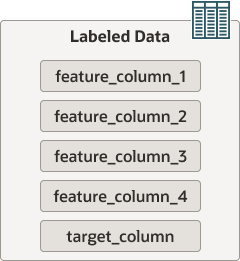MySQL HeatWave User Guide  /  HeatWave ML  /  Preparing Data

## 3.3 Preparing Data

HeatWave ML works with labeled an unlabeled data. Labeled data is used to train and score machine learning models. Unlabelled data is required when generating predictions and explanations.

### Labeled Data

Labeled data has feature columns and a target column (the label), as illustrated in the following diagram:

Figure 3.2 Labeled DataFeature columns contain the input variables used to train the machine learning model. The target column contains ground truth values or, in other words, the correct answers. A labeled dataset with ground truth values is required to train a machine learning model. In the context of this guide, the labeled dataset used to train a machine learning model is referred as the training dataset.

A labeled dataset with ground truth values is also used to score a model (compute its accuracy and reliability). This dataset should have the same columns as the training dataset but with a different set of data. In the context of this guide, the labeled dataset used to score a model is referred as the validation dataset.

### Unlabeled Data

Unlabeled data has feature columns but no target column (no answers), as illustrated below:

Figure 3.3 Unlabeled DataUnlabeled data is required to generate predictions and explanations. It must have exactly the same feature columns as the training dataset but no target column. In the context of this guide, the unlabeled data used for predictions and explanations is referred to as the test dataset. Test data starts as labeled data but the label is removed for the purpose of trialing the machine learning model.

The unseen data that you will eventually use with your model to make predictions is also unlabeled data. Like the test dataset, unseen data must have exactly the same feature columns as the training dataset but no target column.

For examples of training, validation, and test dataset tables and how they are structured, see Example Data, and Section 6.3, “Iris Data Set Machine Learning Quickstart”.

### General Data Requirements

General requirements for HeatWave ML data include the following:

• Each dataset must reside in a single table on the MySQL DB System. HeatWave ML routines such as `ML_TRAIN`, `ML_PREDICT_TABLE`, and `ML_EXPLAIN_TABLE` operate on a single table.

• Tables used with HeatWave ML must not exceed 10 GB, 100 million rows, or 900 columns.

• Table columns must use supported data types. For supported data types and recommendations for how to handle unsupported types, see Section 3.9, “Supported Data Types”.

• NaN (Not a Number) values are not recognized by MySQL and should be replaced by `NULL`.

• The target column in a training dataset for a classification model must have at least two distinct values, and each distinct value should appear in at least five rows. For a regression model, only a numeric target column is permitted.

Note

The `ML_TRAIN` routine ignores columns missing more than 20% of its values and columns with the same value in each row. Missing values in numerical columns are replaced with the average value of the column, standardized to a mean of 0 and with a standard deviation of 1. Missing values in categorical columns are replaced with the most frequent value, and either one-hot or ordinal encoding is used to convert categorical values to numeric values. The input data as it exists in the MySQL database is not modified by `ML_TRAIN`.

### Example Data

Examples in this guide use the Census Income Data Set.

Dua, D. and Graff, C. (2019). UCI Machine Learning Repository [http://archive.ics.uci.edu/ml]. Irvine, CA: University of California, School of Information.

Note

Census Income Data Set examples demonstrate `classification` training and inference. HeatWave ML also supports `regression` training and inference for datasets suited for that purpose. The `ML_TRAIN` `task` parameter defines whether the machine learning model is trained for `classification` or `regression`.

To replicate the examples in this guide, perform the following steps to create the required schema and tables. Python 3 and MySQL Shell are required.

1. Create the following schema and tables on the MySQL DB System by executing the following statements:

``````CREATE SCHEMA heatwaveml_bench;

USE heatwaveml_bench;

CREATE TABLE census_train (
age INT, workclass VARCHAR(255),
fnlwgt INT, education VARCHAR(255),
`education-num` INT,
`marital-status` VARCHAR(255),
occupation VARCHAR(255),
relationship VARCHAR(255),
race VARCHAR(255),
sex VARCHAR(255),
`capital-gain` INT,
`capital-loss` INT,
`hours-per-week` INT,
`native-country` VARCHAR(255),
revenue VARCHAR(255));

CREATE TABLE `census_test` LIKE `census_train`;``````
2. Navigate to the HeatWave ML Code for Performance Benchmarks GitHub repository at https://github.com/oracle-samples/heatwave-ml.

3. Follow the `README.md` instructions to create `census_train.csv` and `census_test.csv` data files. In summary, the instructions are:

1. Install the required Python packages:

``pip install pandas==1.2.3 numpy==1.22.2 unlzw3==0.2.1 sklearn==1.0.2``
2. Download or clone the repository, which includes the census source data and preprpocessing script.

3. Run the `preprocess.py` script to create the `census_train.csv` and `census_test.csv` data files.

``python3 heatwave-ml/preprocess.py --benchmark census``
Note

Do not run the benchmark as instructed in the `README.md` file. The benchmark script removes the schema and data at the end of processing.

4. Start MySQL Shell with the `--mysql` option to open a `ClassicSession`, which is required when using the Parallel Table Import Utility.

``mysqlsh --mysql Username@IPAddressOfMySQLDBSystemEndpoint``
5. Load the data from the `.csv` files into the MySQL DB System using the following commands:

``````MySQL>JS> util.importTable("census_train.csv",{table: "census_train",
dialect: "csv-unix", skipRows:1})

MySQL>JS> util.importTable("census_test.csv",{table: "census_test",
dialect: "csv-unix", skipRows:1})``````
6. Create a validation table:

``````CREATE TABLE `census_validate` LIKE `census_test`;

INSERT INTO `census_validate` SELECT * FROM `census_test`;``````
7. Modify the `census_test` table to remove the target ``revenue`` column:

``ALTER TABLE `census_test` DROP COLUMN `revenue`;``

### Other Example Data Sets

For other example data sets that you can use with HeatWave ML, refer to the HeatWave ML Code for Performance Benchmarks GitHub repository at https://github.com/oracle-samples/heatwave-ml.